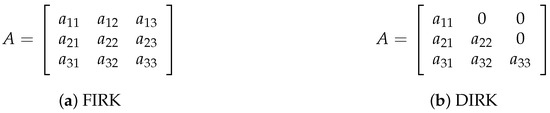Next Article in Journal
Detection of Transcoding from H.264/AVC to HEVC Based on CU and PU Partition Types
Previous Article in Journal
Exploiting Weak Field Gravity-Maxwell Symmetry in Superconductive Fluctuations Regime
Open AccessArticle

# Diagonally Implicit Block Backward Differentiation Formula with Optimal Stability Properties for Stiff Ordinary Differential Equations

byHazizah Mohd Ijam 1andZarina Bibi Ibrahim 1,2,*1
Institute for Mathematical Research, Universiti Putra Malaysia (UPM), Serdang 43400, Selangor, Malaysia
2
Department of Mathematics, Faculty of Science, Universiti Putra Malaysia (UPM), Serdang 43400, Selangor, Malaysia
*
Author to whom correspondence should be addressed.
Symmetry 2019, 11(11), 1342; https://doi.org/10.3390/sym11111342
Received: 20 August 2019 / Revised: 22 September 2019 / Accepted: 26 September 2019 / Published: 1 November 2019
This paper aims to select the best value of the parameter $ρ$ from a general set of linear multistep formulae which have the potential for efficient implementation. The $ρ$ -Diagonally Implicit Block Backward Differentiation Formula ( $ρ$ -DIBBDF) was proposed to approximate the solution for stiff Ordinary Differential Equations (ODEs) to achieve the research objective. The selection of $ρ$ for optimal stability properties in terms of zero stability, absolute stability, error constant and convergence are discussed. In the diagonally implicit formula that uses a lower triangular matrix with identical diagonal entries, allowing a maximum of one lower-upper (LU) decomposition per integration stage to be performed will result in substantial computing benefits to the user. The numerical results and plots of accuracy indicate that the $ρ$ -DIBBDF method performs better than the existing fully and diagonally Block Backward Differentiation Formula (BBDF) methods. View Full-Text
Show FiguresFigure 1

MDPI and ACS Style

Mohd Ijam, H.; Ibrahim, Z.B. Diagonally Implicit Block Backward Differentiation Formula with Optimal Stability Properties for Stiff Ordinary Differential Equations. Symmetry 2019, 11, 1342.